Publicité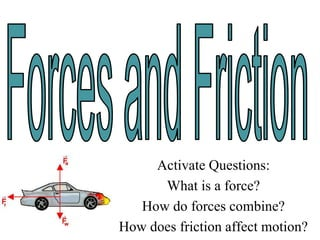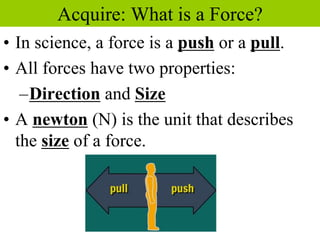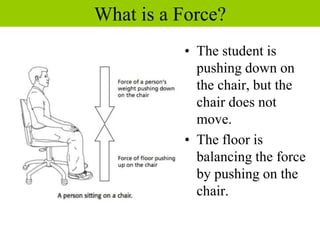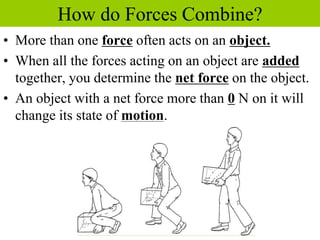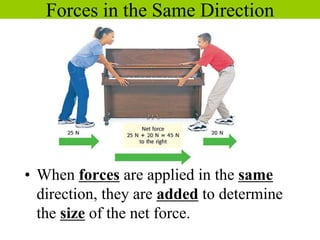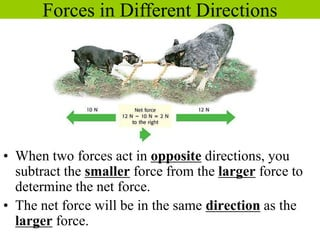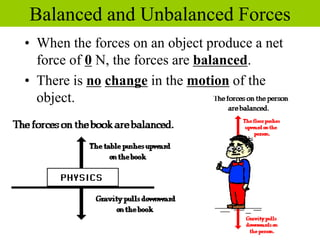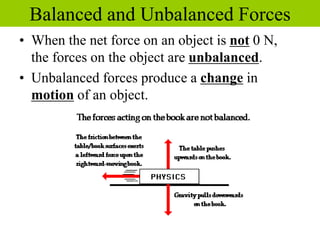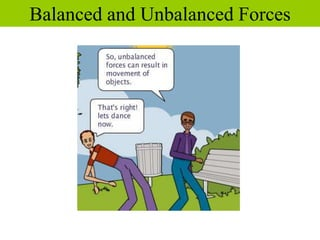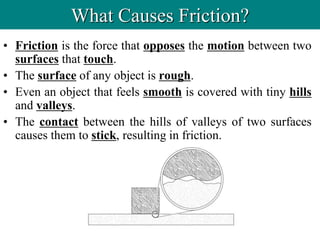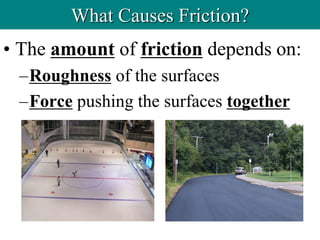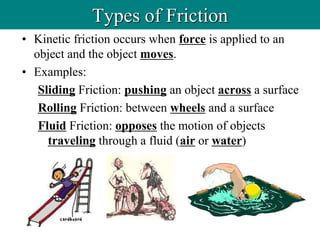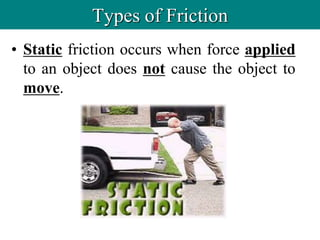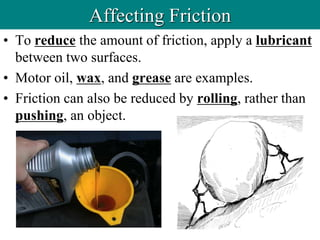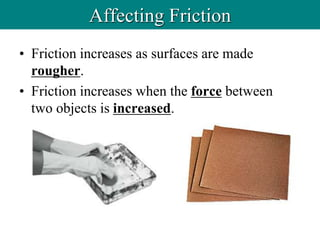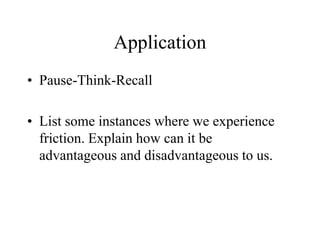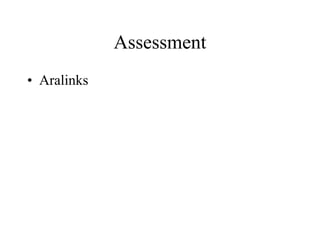1 sur 17
Publicité

### Week 3.ppt

1. Activate Questions: What is a force? How do forces combine? How does friction affect motion?
2. Acquire: What is a Force? • In science, a force is a push or a pull. • All forces have two properties: –Direction and Size • A newton (N) is the unit that describes the size of a force.
3. • The student is pushing down on the chair, but the chair does not move. • The floor is balancing the force by pushing on the chair. What is a Force?
4. • More than one force often acts on an object. • When all the forces acting on an object are added together, you determine the net force on the object. • An object with a net force more than 0 N on it will change its state of motion. How do Forces Combine?
5. Forces in the Same Direction • When forces are applied in the same direction, they are added to determine the size of the net force.
6. • When two forces act in opposite directions, you subtract the smaller force from the larger force to determine the net force. • The net force will be in the same direction as the larger force. Forces in Different Directions
7. • When the forces on an object produce a net force of 0 N, the forces are balanced. • There is no change in the motion of the object. Balanced and Unbalanced Forces
8. • When the net force on an object is not 0 N, the forces on the object are unbalanced. • Unbalanced forces produce a change in motion of an object. Balanced and Unbalanced Forces
9. Balanced and Unbalanced Forces
10. What Causes Friction? • Friction is the force that opposes the motion between two surfaces that touch. • The surface of any object is rough. • Even an object that feels smooth is covered with tiny hills and valleys. • The contact between the hills of valleys of two surfaces causes them to stick, resulting in friction.
11. • The amount of friction depends on: –Roughness of the surfaces –Force pushing the surfaces together What Causes Friction?
12. • Kinetic friction occurs when force is applied to an object and the object moves. • Examples: Sliding Friction: pushing an object across a surface Rolling Friction: between wheels and a surface Fluid Friction: opposes the motion of objects traveling through a fluid (air or water) Types of Friction
13. • Static friction occurs when force applied to an object does not cause the object to move. Types of Friction
14. • To reduce the amount of friction, apply a lubricant between two surfaces. • Motor oil, wax, and grease are examples. • Friction can also be reduced by rolling, rather than pushing, an object. Affecting Friction
15. • Friction increases as surfaces are made rougher. • Friction increases when the force between two objects is increased. Affecting Friction
16. Application • Pause-Think-Recall • List some instances where we experience friction. Explain how can it be advantageous and disadvantageous to us.